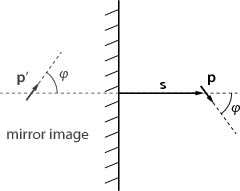10th of September, 2008

1. The electric potential $\varphi$ must satisfy Poisson’s equation.
${\nabla }^{2}\varphi =-\frac{\rho }{{\epsilon }_{0}}$

The spatial charge density can be expressed in terms of the concentration $n$ and charge $q$ of positive and negative ions:

$\rho ={n}_{+}{q}_{+}+{n}_{-}{q}_{-}$

For simplicity, consider the case when ${q}_{+}=-{q}_{-}=q$, and the concentration of both kinds of ions is ${n}_{0}$ far from the colloidal particle.

${n}_{+}={n}_{0}{e}^{-q\varphi ∕kT}\phantom{\rule{2em}{0ex}}{n}_{-}={n}_{0}{e}^{q\varphi ∕kT}$

For $kT\gg q\varphi$ the approximation ${e}^{x}\approx 1+x$ can be used. Thus

$\rho \approx -\frac{2{n}_{0}{q}^{2}\varphi }{kT}.$

Substituting this into Poisson’s equation and using the spherical symmetry of the problem we get

$\frac{{\partial }^{2}\varphi }{\partial {r}^{2}}+\frac{2}{r}\frac{\partial \varphi }{\partial \rho }-\underset{C}{\underbrace{\frac{2{n}_{0}{q}^{2}}{kT}}}\varphi =0.$

This differential equation can be written as

$\frac{{\partial }^{2}\left(r\varphi \right)}{\partial {r}^{2}}-C\left(r\varphi \right)=0,$

whose general solution is $r\varphi ={C}_{1}{e}^{-\sqrt{C}r}+{C}_{2}{e}^{\sqrt{C}r}$. For the potential to be zero at infinity, it is required that ${C}_{2}=0$. When $r\to 0$, the potential must be the same as in the absence of any ions: ${C}_{1}=Q∕4\pi {\epsilon }_{0}$, where $Q$ is the charge of the colloidal particle.

$\varphi \left(r\right)=\frac{1}{4\pi {ϵ}_{0}}\frac{Q}{r}{e}^{-\sqrt{2{n}_{0}{q}^{2}∕kT}r}$

2. The problem can be solved using the method of images. The electric potential inside a conductor is constant, and the field vector near a conducting surface is always perpendicular to the surface. The charges inside the conducting sheet redistribute themselves in such a way that the field created by them balances that component of the dipole’s field which is parallel to the sheet.Figure 1: Using the method of images: the electric field in the right half-space will be the same as a field that would be created by the mirrored dipole ${\stackrel{\to }{p}}^{\prime }$.

Let us imagine that the sheet is removed, and another electric dipole ${\stackrel{\to }{p}}^{\prime }$ is added in such a way that it is the mirror image of $\stackrel{\to }{p}$ with respect to the plane of the sheet, but its direction is reversed (see figure 1). It is easy to see that the electric potential created by $\stackrel{\to }{p}$ and ${\stackrel{\to }{p}}^{\prime }$ together is constant in the mirror plane. We know that the solution of Laplace’s equation is unique if the boundary conditions are fixed. Thus the field created in the right-hand-side half-space by the charges on the conducting sheet must be the same as the field created by a mirror dipole ${\stackrel{\to }{p}}^{\prime }$ in the absence of the sheet. Now all we need to do is find the torque acting on $\stackrel{\to }{p}$ in such a field.

The electric potential created by a dipole ${\stackrel{\to }{p}}^{\prime }$ is

$V\left(\stackrel{\to }{r}\right)=\frac{1}{4\pi {\epsilon }_{0}}\frac{{\stackrel{\to }{p}}^{\prime }\cdot \stackrel{\to }{r}}{{r}^{3}}.$

The electric field vector is

$\stackrel{\to }{E}\left(\stackrel{\to }{r}\right)=-\nabla V=\frac{1}{4\pi {\epsilon }_{0}}\frac{3\left({\stackrel{\to }{p}}^{\prime }\cdot \stackrel{\to }{r}\right)\stackrel{\to }{r}-{r}^{2}{\stackrel{\to }{p}}^{\prime }}{{r}^{5}},$

and the potential energy of a dipole $\stackrel{\to }{p}$ in this field is

$W=-\stackrel{\to }{E}\cdot \stackrel{\to }{p}=\frac{1}{4\pi {\epsilon }_{0}}\frac{{r}^{2}\left(\stackrel{\to }{p}\cdot {\stackrel{\to }{p}}^{\prime }\right)-3\left(\stackrel{\to }{r}\cdot \stackrel{\to }{p}\right)\left(\stackrel{\to }{r}\cdot {\stackrel{\to }{p}}^{\prime }\right)}{{r}^{5}}.$

Let $\theta$ and ${\theta }^{\prime }$ denote the angles between $\stackrel{\to }{r}$, and the vectors $\stackrel{\to }{p}$ and $\stackrel{\to }{p}$’, respectively. If both $\stackrel{\to }{p}$ and ${\stackrel{\to }{p}}^{\prime }$ have the same magnitude, then the potential energy can be written as

$W=\frac{{p}^{2}}{4\pi {\epsilon }_{0}}\frac{cos\left(\theta +{\theta }^{\prime }\right)-3cos\theta \phantom{\rule{3.26212pt}{0ex}}cos{\theta }^{\prime }}{{r}^{3}}.$

The magnitude of the torque acting on the dipole $\stackrel{\to }{p}$ is just

$T=-\frac{\partial W}{\partial \theta }=-\frac{{p}^{2}}{4\pi {\epsilon }_{0}}\frac{-sin\left(\theta +{\theta }^{\prime }\right)+3sin\theta \phantom{\rule{3.26212pt}{0ex}}cos{\theta }^{\prime }}{{r}^{3}}.$

Let us apply this result to the dipole and its mirror image. We have $r=2s$ and $\theta ={\theta }^{\prime }=\phi$, so

If the dipole is free to rotate, it will adopt one of the positions where the torque is 0, i.e. $\phi =0$, $\phi =\pi ∕2$, or $\phi =\pi$. Of these three, $\phi =\pi ∕2$ is an unstable equilibrium, so the dipole will be perpendicular to the plane.

3. We have essentially a one dimensional problem, because $A\gg d$. We can write Poisson’s equation for $V$:
$\frac{{d}^{2}V\left(x\right)}{d{x}^{2}}=-\frac{\rho \left(x\right)}{{\epsilon }_{0}}$

In a steady state the current density $I∕A=\rho \left(x\right)v\left(x\right)$ between the plates is constant. Here $v\left(x\right)$ denotes the velocity of the electrons when they are at distance $x$ from the cathode. The electrons gain their kinetic energy from the electric field, so ${E}_{kinetic}=m{v}^{2}∕2=eV\left(x\right)$, where $e$ is the elementary charge. Using these two relations we find that $\rho \left(x\right)=\left(I∕A\right)\sqrt{m}∕\sqrt{2eV\left(x\right)}$. Substituting this result into Poisson’s equation,

$\frac{{d}^{2}V\left(x\right)}{d{x}^{2}}\sqrt{V\left(x\right)}=-\frac{I\sqrt{m}}{A{\epsilon }_{0}\sqrt{2e}}=C$

Note that $C>0$ because the physical current is flowing from the anode to the cathode: $j<0$. We seek the solution of this differential equation in the form $V\left(x\right)=\beta {x}^{\alpha }$. Substituting this back into the equation we find that $\alpha =4∕3$ and $\beta ={\left(9C∕4\right)}^{2∕3}$. This is not a general solution as it does not contain any arbitrary constants but it satisfies the boundary conditions of the problem: $V\left(0\right)=0$ and ${\frac{dV}{dx}|}_{x=0}=0$ (the electric field is $0$ at the cathode), so we need seek no further. The final results are

$V\left(x\right)={\left(\frac{81}{32}\frac{{I}^{2}m}{{A}^{2}{\epsilon }_{0}^{2}e}\right)}^{1∕3}{x}^{4∕3}$

$v\left(x\right)={\left(\frac{9}{2}\frac{Ie}{A{\epsilon }_{0}m}\right)}^{1∕3}{x}^{2∕3}$

$\rho \left(x\right)={\left(\frac{2}{9}\frac{{I}^{2}{\epsilon }_{0}m}{{A}^{2}e}\right)}^{1∕3}{x}^{-2∕3}$

Putting $x=d$ to find ${V}_{0}$, we get

$I=\frac{4}{9}\sqrt{\frac{2e}{m}}\frac{A{\epsilon }_{0}}{{d}^{2}}{V}_{0}^{3∕2}$

Back to the main page